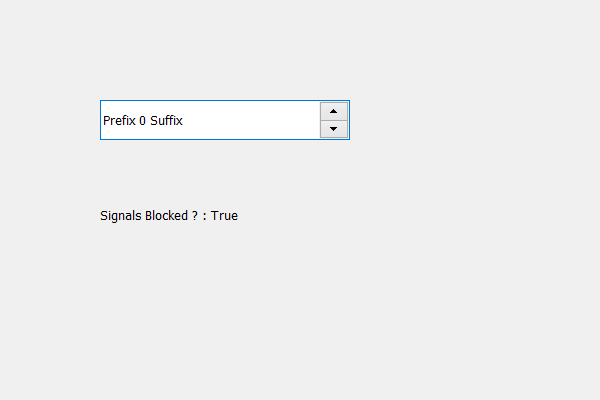GFG App
Open AppBrowser
Continue

# PyQt5 QSpinBox – Checking if signals are blocked or not

In this article we will see how we can check if the signals are blocked or not of the spin box, blocking signals means the connected methods with the spin box will not receive the signals for example when we add action to it when its value changes that action will not work. Signals emitted while being blocked are not buffered. In order to block signals we use `blockSignals` method.

In order to do this we use signalsBlocked method.

Syntax : spin_box.signalsBlocked()

Argument : It takes no argument

Return : It returns None

Below is the implementation

 `# importing libraries ` `from` `PyQt5.QtWidgets ``import` `*`  `from` `PyQt5 ``import` `QtCore, QtGui ` `from` `PyQt5.QtGui ``import` `*`  `from` `PyQt5.QtCore ``import` `*`  `import` `sys ` ` `  ` `  `class` `Window(QMainWindow): ` ` `  `    ``def` `__init__(``self``): ` `        ``super``().__init__() ` ` `  `        ``# setting title ` `        ``self``.setWindowTitle(``"Python "``) ` ` `  `        ``# setting geometry ` `        ``self``.setGeometry(``100``, ``100``, ``600``, ``400``) ` ` `  `        ``# calling method ` `        ``self``.UiComponents() ` ` `  `        ``# showing all the widgets ` `        ``self``.show() ` ` `  `    ``# method for widgets ` `    ``def` `UiComponents(``self``): ` `        ``# creating spin box ` `        ``self``.spin ``=` `QSpinBox(``self``) ` ` `  `        ``# setting geometry to spin box ` `        ``self``.spin.setGeometry(``100``, ``100``, ``250``, ``40``) ` ` `  `        ``# setting prefix to spin ` `        ``self``.spin.setPrefix(``"Prefix "``) ` ` `  `        ``# setting suffix to spin ` `        ``self``.spin.setSuffix(``" Suffix"``) ` ` `  `        ``# blocking signals of the spin box ` `        ``self``.spin.blockSignals(``True``) ` ` `  `        ``# creating a label ` `        ``self``.label ``=` `QLabel(``"Label "``, ``self``) ` ` `  `        ``# setting geometry to the label ` `        ``self``.label.setGeometry(``100``, ``200``, ``300``, ``30``) ` ` `  `        ``# checking if signals are blocked or not ` `        ``check ``=` `self``.spin.signalsBlocked() ` ` `  `        ``# setting text to the label ` `        ``self``.label.setText(``"Signals Blocked ? : "` `+` `str``(check)) ` ` `  ` `  ` `  `# create pyqt5 app ` `App ``=` `QApplication(sys.argv) ` ` `  `# create the instance of our Window ` `window ``=` `Window() ` ` `  `# start the app ` `sys.exit(App.``exec``()) `

Output :My Personal Notes arrow_drop_up